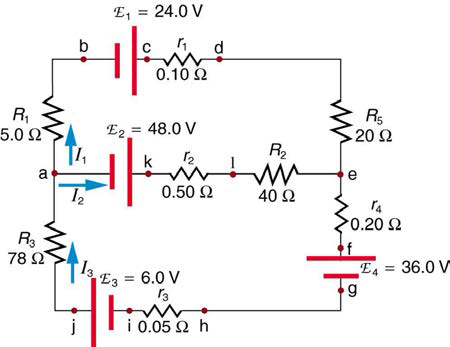×
Get Full Access to College Physics - 1 Edition - Chapter 21 - Problem 38pe
Get Full Access to College Physics - 1 Edition - Chapter 21 - Problem 38pe

×

# Find the currents flowing in the circuit in Figure 21.52.ISBN: 9781938168000 42

## Solution for problem 38PE Chapter 21

College Physics | 1st Edition

• Textbook Solutions
• 2901 Step-by-step solutions solved by professors and subject experts
• Get 24/7 help from StudySoup virtual teaching assistantsCollege Physics | 1st Edition

4 5 1 408 Reviews
22
3
Problem 38PE

Find the currents flowing in the circuit in Figure $$21.52$$. Explicitly show how you follow the steps in the Problem-Solving Strategies for Series and Parallel Resistors.Figure $$21.52$$

Equation Transcription:Text Transcription:

21.52

Step-by-Step Solution:
Step 1 of 3

Solution 38PE

Apply the formula of node at point a,

I3 =I1 + I2

I1 + I2 -I3  = 0…… (1)

Apply the loop rule in loop aklefghija as follows,

40.5 I2 + 78.25 I3  = 18…… (2)

Apply the loop rule in loop abcdelka as follows,

25.1 I1 + 50.1 I2  = 24…… (3)

Now, substitute the value of  I3 from equation (1) into equation (2), we get

25.1 I1 + 78.25 ( I1 + I2 ) = 18

25.1 I1 + 78.25 I1 + 78.25 I2 = 18

103.35I1 + 78.25I2 = 18…… (4)

Now, simplify the equation (4) and equation (3) and by solving the equations the value of currents is, I1 = 0.54A,  I2 = -0.2057A and .I3 = 0.3365A

the current flowing in the circuit is I1 = 0.54A, I2 = -0.2057A and I3 = 0.3365A

Step 2 of 3

Step 3 of 3

##### ISBN: 9781938168000

The full step-by-step solution to problem: 38PE from chapter: 21 was answered by , our top Physics solution expert on 07/07/17, 04:39PM. The answer to “?Find the currents flowing in the circuit in Figure $$21.52$$. Explicitly show how you follow the steps in the Problem-Solving Strategies for Series and Parallel Resistors. Figure $$21.52$$Equation Transcription:Text Transcription:21.52” is broken down into a number of easy to follow steps, and 30 words. College Physics was written by and is associated to the ISBN: 9781938168000. Since the solution to 38PE from 21 chapter was answered, more than 2291 students have viewed the full step-by-step answer. This full solution covers the following key subjects: Circuit, currents, explicitly, figure, Find. This expansive textbook survival guide covers 34 chapters, and 3125 solutions. This textbook survival guide was created for the textbook: College Physics , edition: 1.

Unlock Textbook Solution